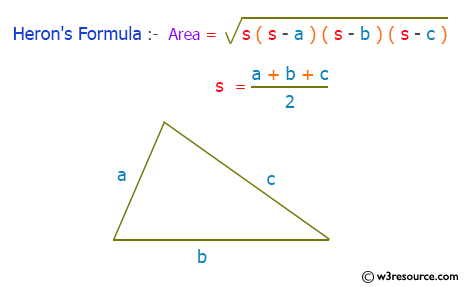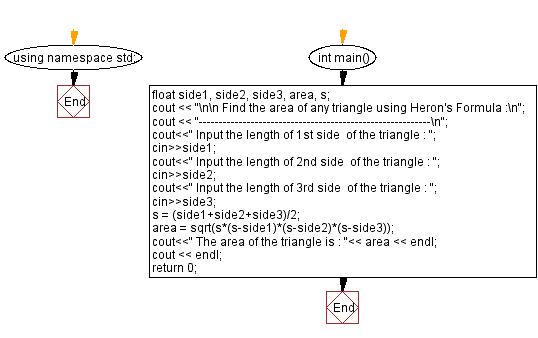﻿ C++ : Find the area of any triangle using Herons Formula

# C++ Exercises: Find the area of any triangle using Heron's Formula

## C++ Basic: Exercise-18 with Solution

Write a C++ program to find the area of any triangle using Heron's formula.

Pictorial Presentation:Sample Solution:

C++ Code :

``````#include <iostream>
#include <math.h>
using namespace std;

int main()
{
float side1, side2, side3, area, s;
cout << "\n\n Find the area of any triangle using Heron's Formula :\n";
cout << "----------------------------------------------------------\n";
cout<<" Input the length of 1st side  of the triangle : ";
cin>>side1;
cout<<" Input the length of 2nd side  of the triangle : ";
cin>>side2;
cout<<" Input the length of 3rd side  of the triangle : ";
cin>>side3;
s = (side1+side2+side3)/2;
area = sqrt(s*(s-side1)*(s-side2)*(s-side3));
cout<<" The area of the triangle is : "<< area << endl;
cout << endl;
return 0;
}
``````

Sample Output:

``` Find the area of any triangle using Heron's Formula :
----------------------------------------------------------
Input the length of 1st side  of the triangle : 5
Input the length of 2nd side  of the triangle : 5
Input the length of 3rd side  of the triangle : 5
The area of the triangle is : 10.8253
```

Flowchart:C++ Code Editor:

What is the difficulty level of this exercise?

﻿

## C++ Programming: Tips of the Day

Why is there no std::stou?

The most pat answer would be that the C library has no corresponding "strtou", and the C++11 string functions are all just thinly veiled wrappers around the C library functions: The std::sto* functions mirror strto*, and the std::to_string functions use sprintf.

Ref: https://bit.ly/3wtz2qA

We are closing our Disqus commenting system for some maintenanace issues. You may write to us at reach[at]yahoo[dot]com or visit us at Facebook